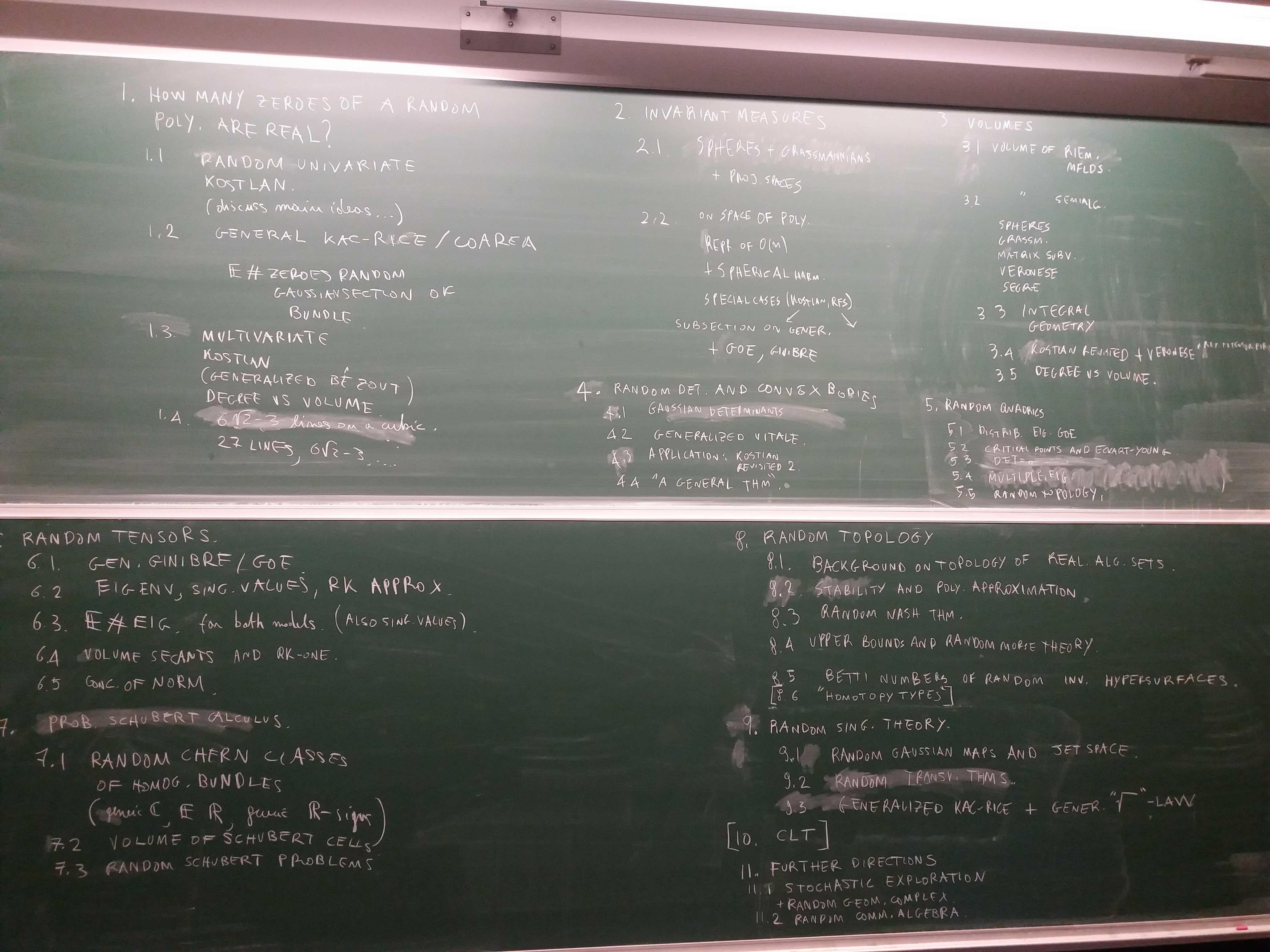Antonio Lerario and I have started writing a book titled

The file under this link is the most recent, but still work in progress.
The last update was on July 8, 2019.

Here is the list of chapters we are thinking of.

1. How many zeros of a random polynomial are real?

2. Counting formulas

3. Volume and degree

4. Topology of random submanifolds

5. Invariant measures

6. Random quadrics

7. Random tensors

8. Probabilistic Schubert calculus

9. Singularity theory

10. Central limit theorems

11. Further directions